# Adding a Calculated multichoice question in the Moodle Question Bank

In the Moodle 2.3 Question Bank, Calculated questions can be set up as multichoice questions. For more information on setting up a Calculated Question click here. The difference between the Calculated Question and the Calculated Multichoice Question is that the calculated question has a text box to input the answer; whereas, the multichoice question has options to select the correct answer. The following will walk through the steps in creating a multichoice question.

### Creating a Calculated multichoice question in the Moodle Question Bank

2. Navigate to Front page settings > Question bank > Questions in the Settings section on the left..
3. Click Create a new question. When the Choose a question type to add box pops up, select the Calculated multichoice radio button and click Next.

4.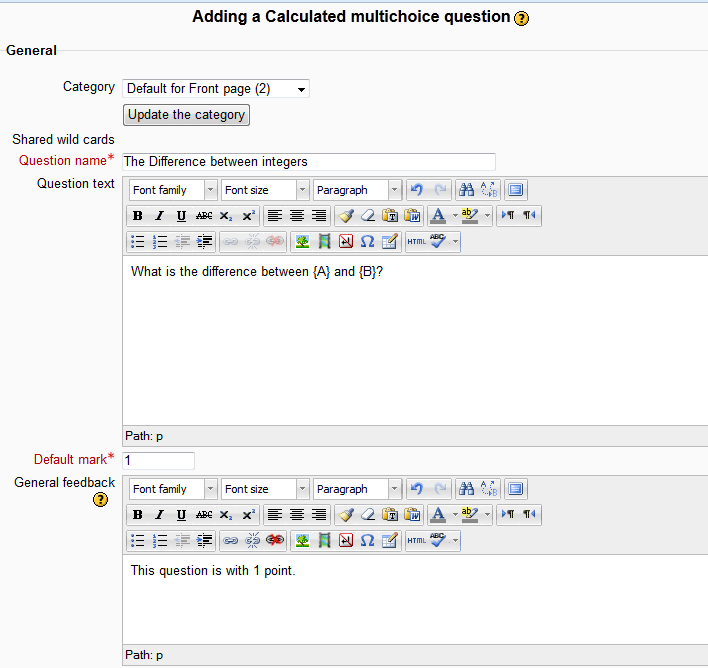In the Adding a Calculated multichoice question page, Fill out the General section. This will be the same as what was done in the Inserting a Calculated question in the Moodle Question Bank tutorial.

5.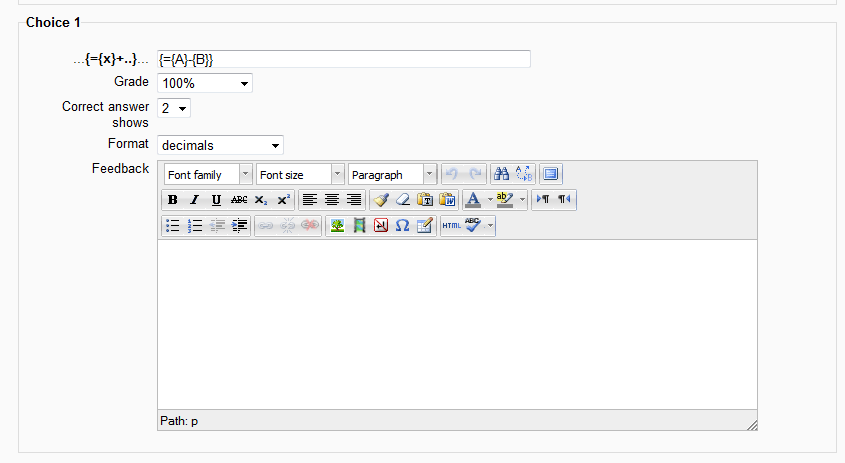Next fill out the Choices for each answer. One choice will be the correct choice and the others will be incorrect. So for Choice 1 the formula will give the correct answer. Choice 2, Choice 3, Choice 4and so forth will contain the incorrect answer. In this tutorial, the question will be:

What is the difference between {A} and {B}?

The following table explains the values necessary for the question to be set up appropriately.

…{={x}+..}… type the formula for the correct answer. The format will basically be in curly brackets with the = in it. For example, in the current question “What is the difference between {A} and {B}?” the correct formula would be {={A}-{B}}. This setting is to display precisely the Answer number of decimal or significant figure. This will display text when the student answers the question..

6.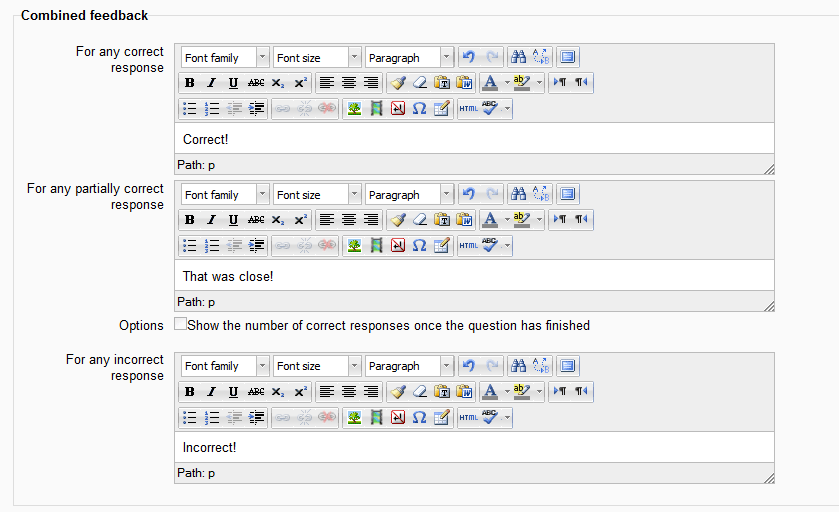In the Combined feedback section, fill in the response that the student will see when they answer the question. Correct, Partially correct, and Incorrect responses can be set here. When the question parameters are set click Save changes.

7.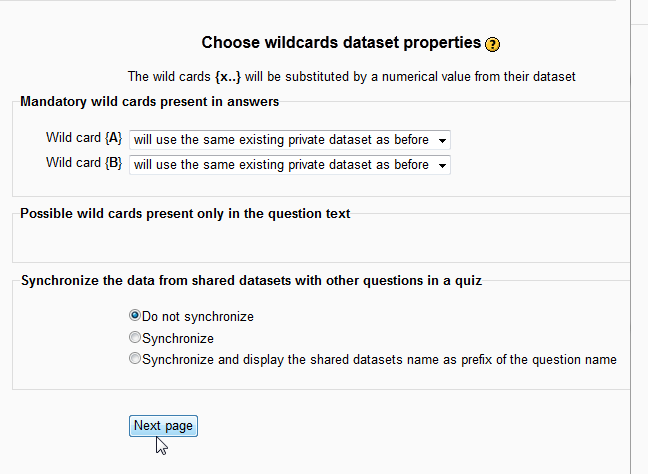On the Choose Wildcards dataset properties page click next. (This section will be discussed in detail in a different article.)

8.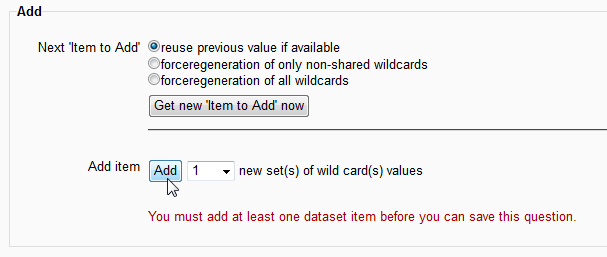On the Edit the wildcards datasets page, where the Add section is located, make sure to click Add.

Important! . If the dataset item is not added, there will be an error message stating:
You must add at least one dataset item before you can save this question.

Once the dataset is added, click Save changes. (This section will be discussed in detail in a different article.)

9.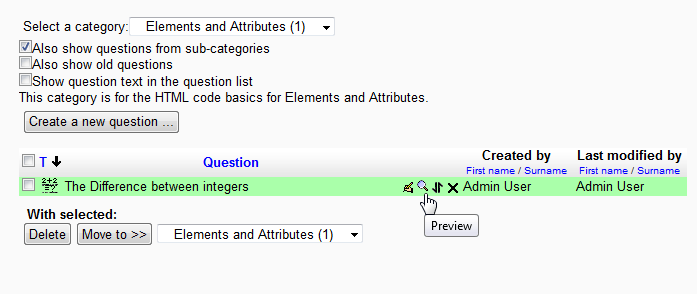Now the question will show in the Question bank. Click the Preview link to preview the question.

10.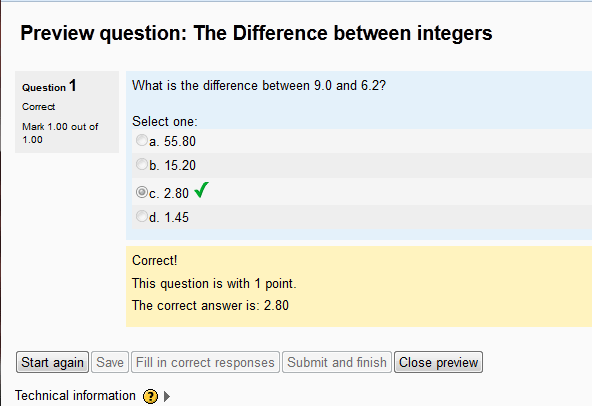The when the question is answered it should look like the following snapshot. Congratulations! the Calculated Multichoice question was created.

This concludes the article on Creating a Calculated multichoice question. Setting up Wildcards and Datasets are an essential part of the Calculated Question. Please check the next article on How to Create Wildcards and datasets in Moodle. Please see the Working with the Question Bank course section for more articles on the Question Bank. Also, visit Working with the calculated question Tolerance in Moodle 2.3 to learn how to configure the Answer Tolerance for Calculated questions.InMotion Hosting Contributor Content Writer

InMotion Hosting contributors are highly knowledgeable individuals who create relevant content on new trends and troubleshooting techniques to help you achieve your online goals!

More Articles by InMotion Hosting